# Barth Sextic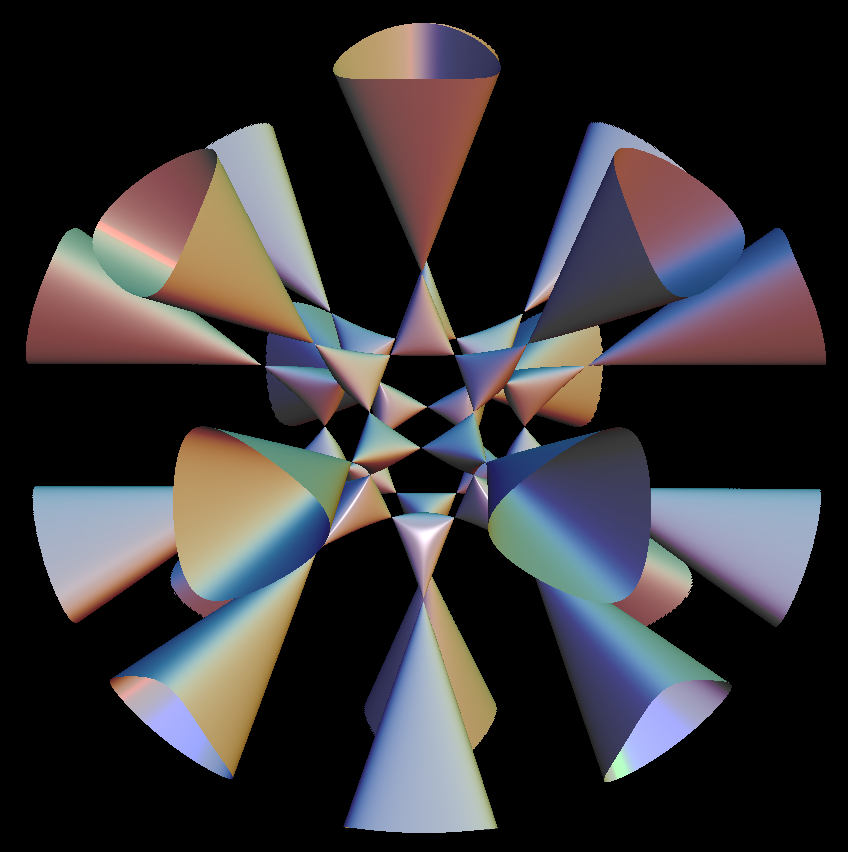barth sextic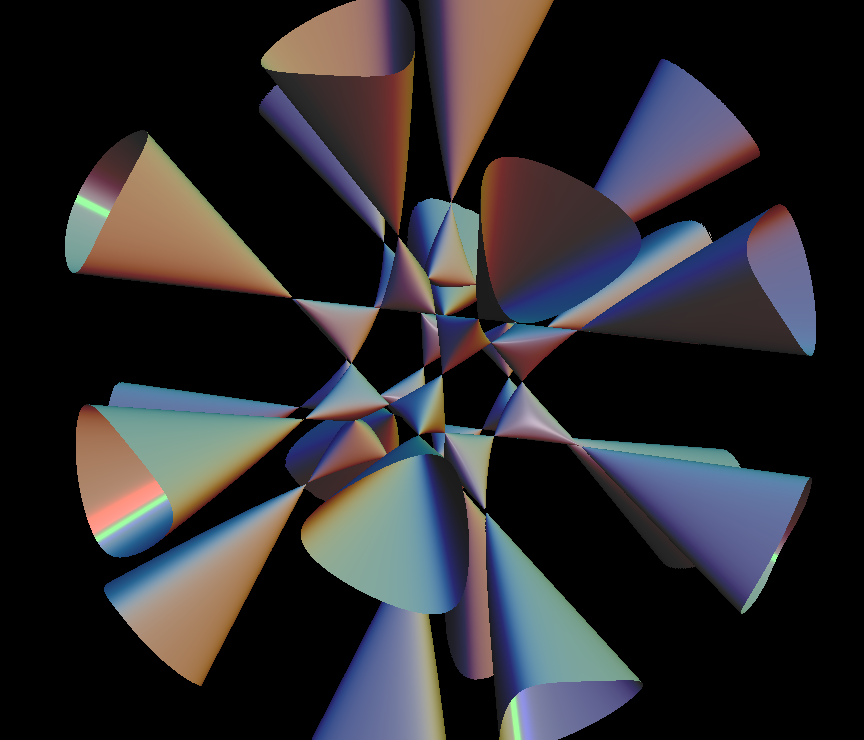This complicated surface is a level surface of a polynomial that has only degree 6. No other degree 6 polynomial with as many conical singularities is known.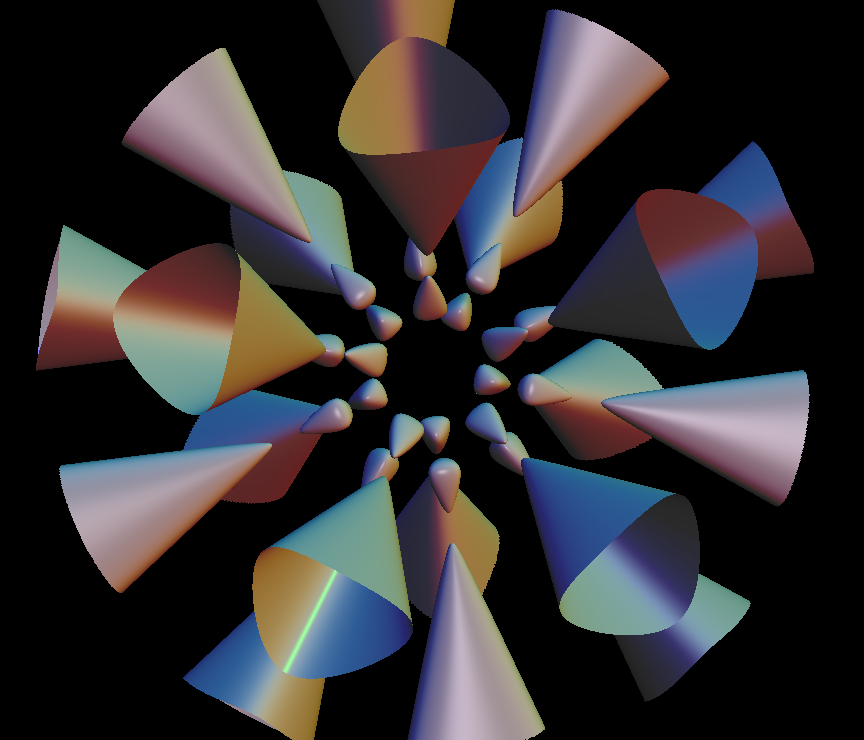Observe the beautiful icosahedral symmetry of the level surfaces in this animation. The transition from the separated pieces through the singular level to the connected levels is called a Morse transition.

Morse Theory

This theory says:

If you have on a compact differentiable manifold M a differentiable function

f : M ⟶ R

such that at every critical point p (i.e. grad f(p) = 0 ) the (symmetric) matrix of second partial derivatives has no 0 eigenvalue, then f is called a Morse function.

The topology of the sublevels of f (i.e. f^{-1} ( [min(f), x] ) ) changes only if x is a critical value (i.e. there exists p with f(p) = x and grad f(p) = 0). The way the topology changes, is determined by the index of the matrix of second partial derivatives at the critical point p.

Our animation sequence shows an example of this change of topology.

For more detail, see Morse theory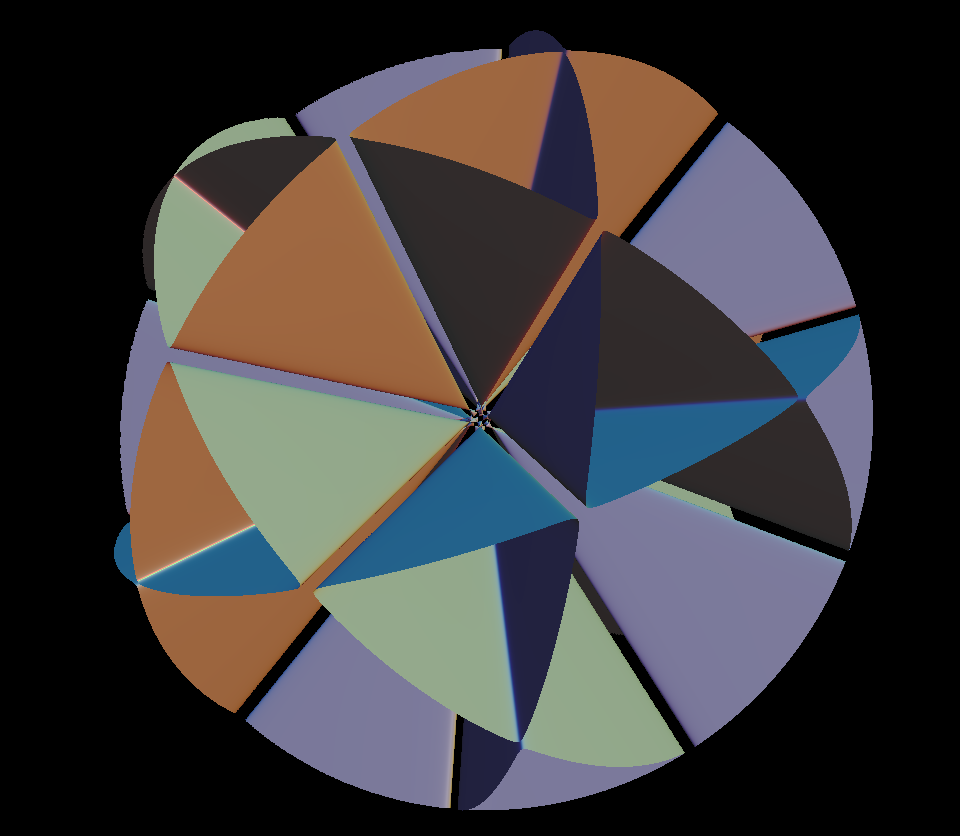Barth Sextic, when looked from far away. It has the symmetry of icosidodecahedron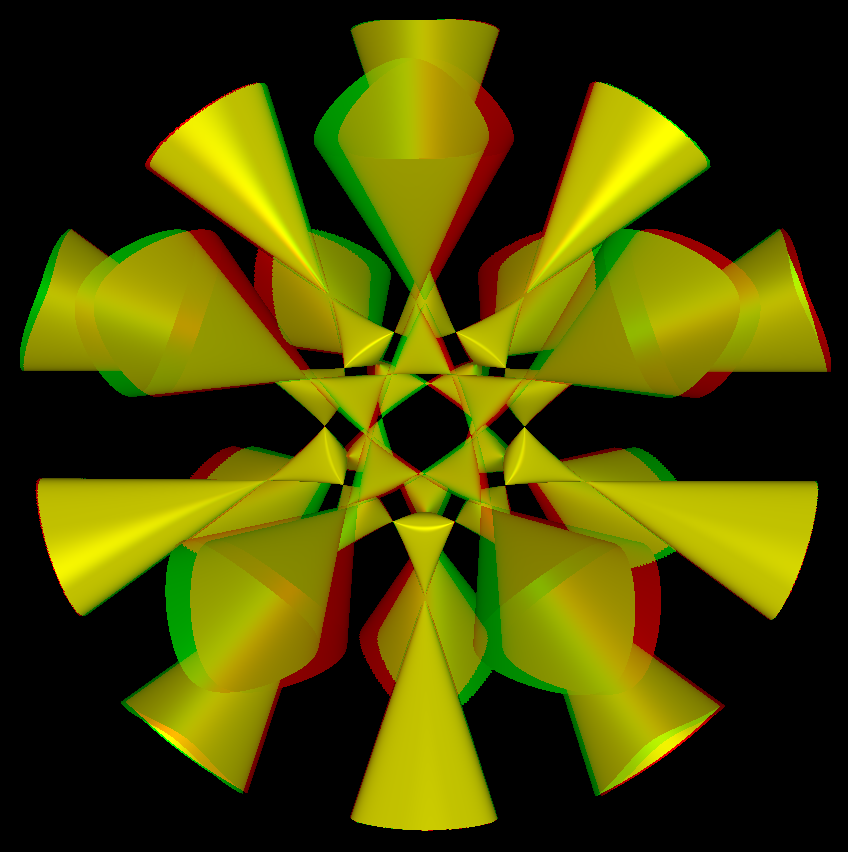barth sextic anaglyph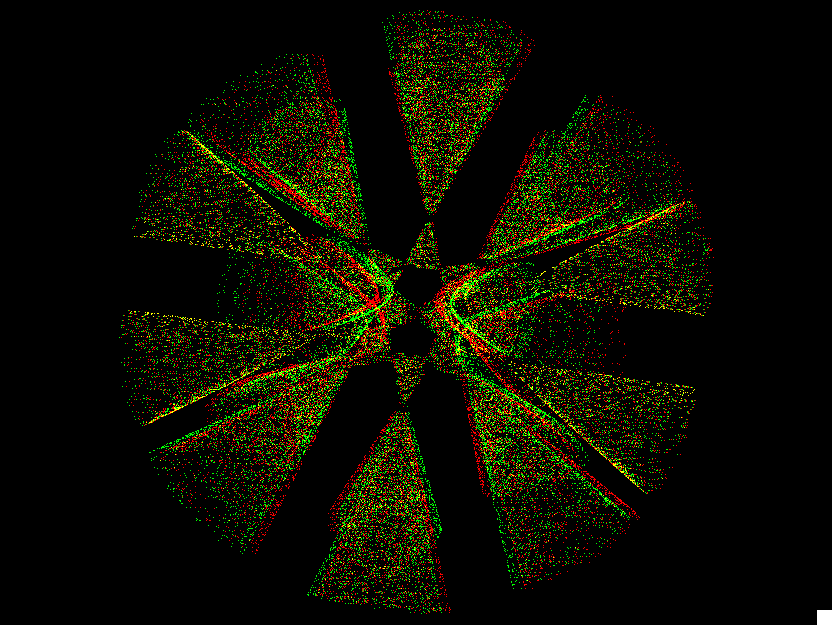barth sextic st

Barth Sextic is a algebraic surface of degree 6. Its formula is:

4*(ϕ^2*x^2-y^2)*(ϕ^2*y^2-z^2)*(ϕ^2*z^2-x^2)-(1+2*ϕ)*(x^2+y^2+z^2-w^2)^2 * w^2 == 0

where ϕ is the golden ratio (ϕ = (1 + Sqrt)/2 ≈ 1.61803) and w is the parameter.

Get red/blue stereo glasses from amazon

ImplicitAlgebraic.pdf